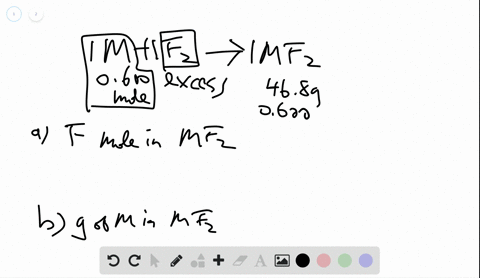🎉 The Study-to-Win Winning Ticket number has been announced! Go to your Tickets dashboard to see if you won! 🎉View Winning Ticket### A sample of 0.600 $\mathrm{mol}$ of a metal M rea…

04:37University of Toronto
Problem 45

# A chloride of silicon contains 79.1 mass $\% \mathrm{Cl.}$ (a) What is the empirical formula of the chloride? If the molar mass is $269 \mathrm{g} / \mathrm{mol},$ what is the molecular formula?

## Discussion

You must be signed in to discuss.

## Video Transcript

So we have a core off second, and from it here, we know that there's every 79.1 mass percentage off. Ah, Corinne. So what would be the empirical formula? Okay, so from here, we know that we're 79.1% of Corinne, and then the west of the percentage should be corresponding to seek. So we're 12.20 point nine percents. They can really just a co write off seconds only of speaking and call, right? So the and people for us, we have to file the racial wishing to level most so soon. We have one dragged graham off our substance. So we're 79.1 Grandma Corinne. And also where 24 9 graham off thinking. We just need to convert that to limbo most. So we have 79.1 off Korean divided by 35 145 more than master, which we would find us ever in 2.23 most. And then for seeker, we have ah, 20 point anagram. T y by, um Ah, the moon. A mass off. Ah, taken. So you have 0.744 and then we're going to simplify this way. Show by divide everything by the smallest number. So we do everything by. Ah, same soup points are settles awful for So we will have roughly around 2.99 to 1 or equal, um 23 to 1. So we would have seek on one thing home with three core. Right? And isn't empirical formula. Okay, so we know that I'm fickle. Foreign. There s I c l three and ah, more than mass off this. Ah, and pickle fornari could calculate from the pr disable. So if we have 28 points here over nine plus 35.45 times three were if we, um Korean. So we should be able to find us roughly around 134.44 Again. We just want to find them multiple off the calculable, The mask. Tiu the, um Okay, what we were doing multiple off our every call formula to keep our molecule for more the mass. So we just take our, um, ocular move from UMAss over there. Empirical bore the mass, so we should be able to find out the motherboard. That's where I've run into this. This is too time war than the Amphicar Fall and Vic Oh, well, the mask. So that's why we're sl sorry as once you see our sakes at asked. Ah, molecular formula.